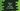# Python factorial of a number : Three different ways to do it## Find factorial of a number using a for loop, while loop and using recursion in python :

In this python programming tutorial, we will learn how to find the factorial of a number programmatically. The program will ask the user to enter a number, it will find the factorial and print it on the console.

### What is factorial of a number :

Factorial of a number is the multiplication of all the numbers from 1 to that number itself e.g. the factorial of 3 is 1 * 2 * 3 i.e. 6.

To find the factorial of a number in python, we can either use one loop or a recursive method. Following examples will explain you properly how to find the factorial of a number in python :

``````# Method 1 : using for loop

def factorialUsingForLoop(n):
fact = 1
for i in range(1,n+1):
fact=fact*i

print('Factorial of the number %d is %d'%(n,fact))

if __name__== "__main__":
factorialUsingForLoop(4)

#Method 2 : using while loop

def factorialUsingWhileLoop(n):
fact = 1
while(n>1):
fact = fact*n
n = n - 1

print('Factorial is %d'%(fact))

if __name__== "__main__":
factorialUsingWhileLoop(4)

#Method 3 : using recursion

def factorialUsingRecursion(n):
if (n == 1):
return 1
else :
return n* factorialUsingRecursion(n-1)

if __name__== "__main__":
print("factorial is ",factorialUsingRecursion(4))``````

### Method 1: Find the factorial of a number using a for loop in python :

Using a for loop, we can iterate from 1 to that specific number we want to find out the factorial. On each iteration of the loop, we will multiply the_ final result_ variable with the_ current iteration count. The first value of the final result variable is _1. Our program will update this value on each iteration of the loo. So, after the loop will exit, the final result will hold the factorial of that number.

In the example above :

1. factorialUsingForLoop method is used to find out the factorial of a number. This method takes one number as its argument. It calculates the factorial for that number and prints the result to the user.
2. Variable ’fact’ is used to hold the final factorial value.
3. Using one ‘for-loop’, we are iterating from 1 to the number ‘n’. Variable ‘i’ is used in this loop. On each iteration of the loop, we are multiplying the current value of the ‘i’ with the ’fact’ variable.
4. Finally, print out the factorial to the user.

### Method 2: using a python while loop :

Similar to the above program, we can use one ’while’ loop to find out the factorial. The process is the same. The only difference is that we are using one ’while’ loop instead of a ’for loop‘.

factorialUsingWhileLoop’ method is used to find out the factorial using a while loop. Similar to the above program, the variable ’fact’ is used to hold the final factorial value. The while loop will run until the value of ‘n’ is greater than ‘1’. On each iteration of the loop, we are decreasing the value of ‘n’ by ’1’. This loop will exit when the value of ’n’ will be ’0’. We are printing the factorial value when it ends.

### Method 3: using Recursion to find factorial of a number in Python:

Recursion means the same function will be called recursively. To find factorial of a number, we can also use a recursive approach like below :

In this example, factorialUsingRecursion is used to find out the factorial. This method returns the factorial of a number. It is a recursive method.

### Conclusion :

In this tutorial, we have learned how to find out the factorial of a number in python. We have learned how to calculate the factorial using three different methods. Try to run the examples shown above and drop one comment below if you have any queries.

__ View on Github